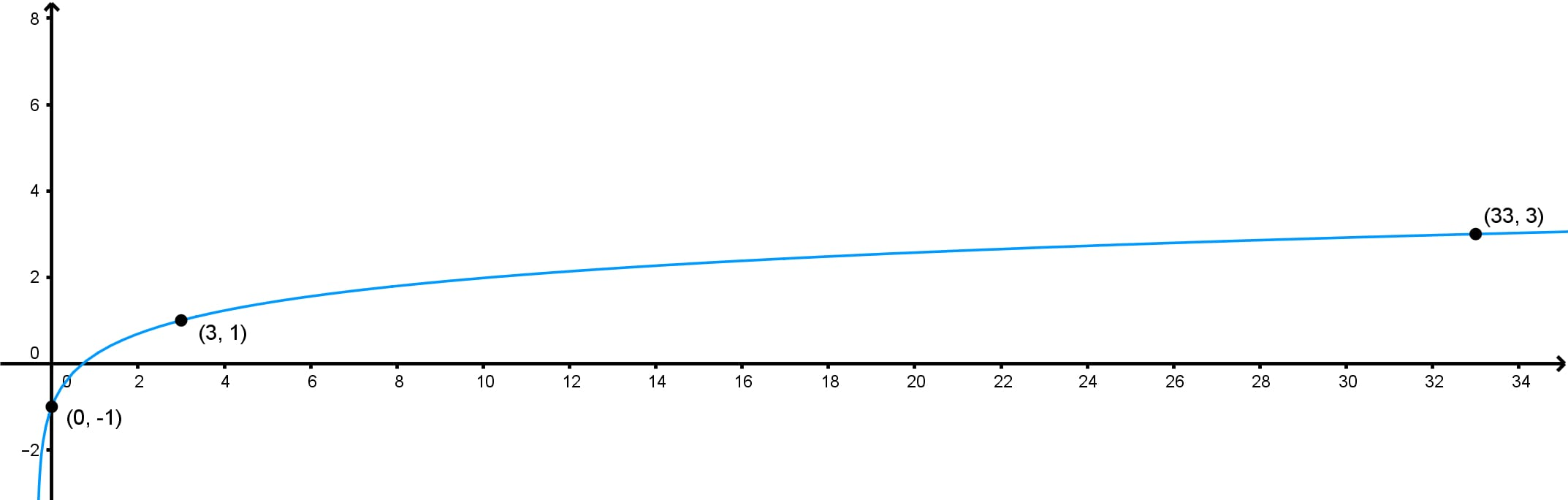# Finding a logarithmic function given its graph#### All in One Place

Everything you need for better grades in university, high school and elementary.#### Learn with Ease

Made in Canada with help for all provincial curriculums, so you can study in confidence.#### Instant and Unlimited Help

0/1
##### Intros
###### Lessons
1. Introduction to finding logarithmic function given its graph

i. What is a logarithmic function?

ii. How can we determine the equation of a logarithmic graph?

0/8
##### Examples
###### Lessons
1. Determining the Equation of an Exponential Function Given Its Graph

Determine an exponential function in the form $y = \log_{b} x$ with the given graph.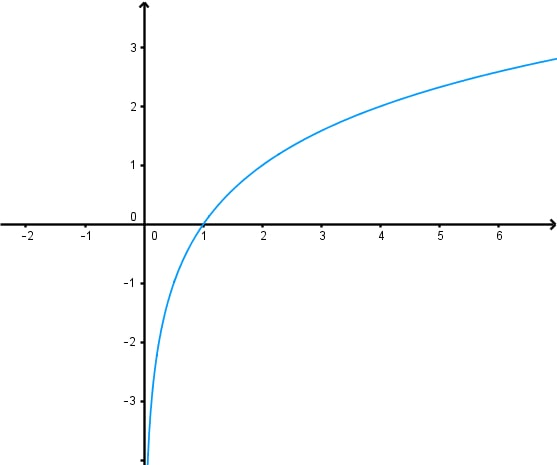1. Determining the Equation of a Transformed Logarithmic function given its Graph

Determine a logarithmic function in the form $y = \log_{2} (x + b) + c$ for each of the given graphs.

1.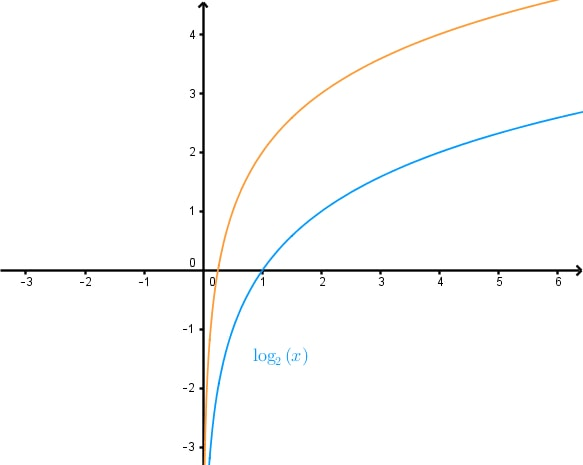2.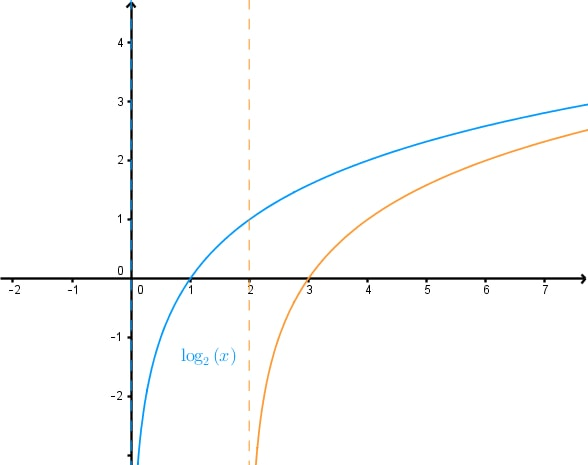2. Determining the Equation of a Transformed Logarithmic function given its Graph - Continued

Determine a logarithmic function in the form $y = A \log_{2} (Bx)$ for each of the given graphs.

1.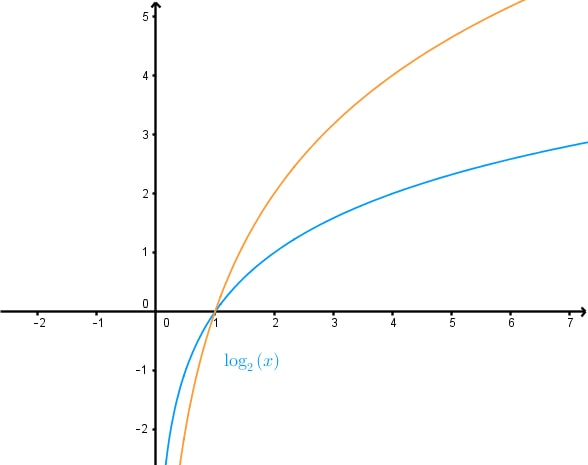2.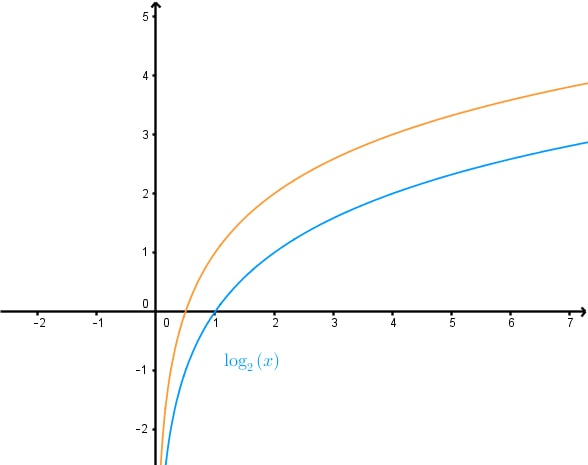3.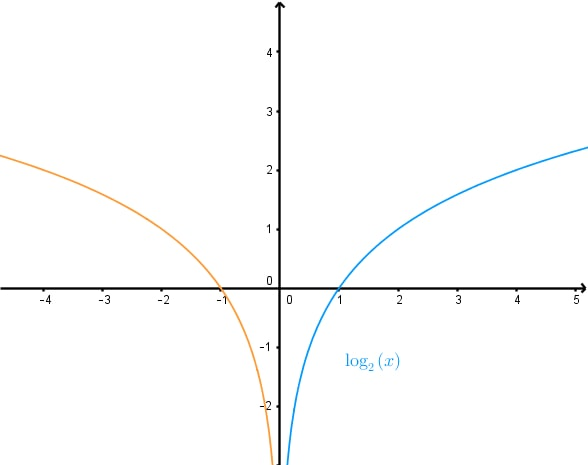4.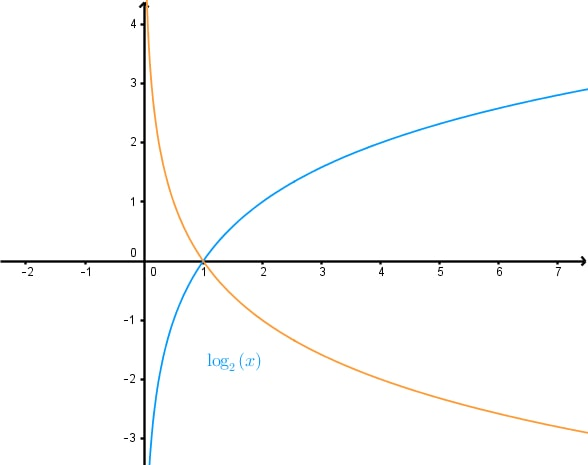3. Determining the Equation of a Logarithmic Graph With Multiple Transformations

Determine a logarithmic function in the form $y = A \log (Bx+1)+C$ for each of the given graphs.